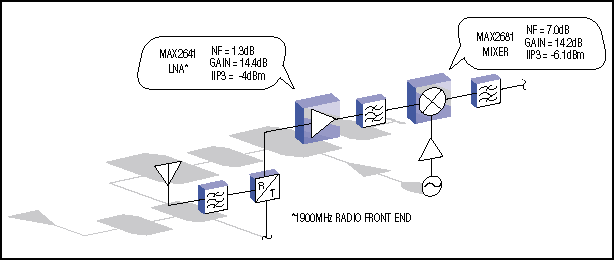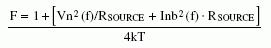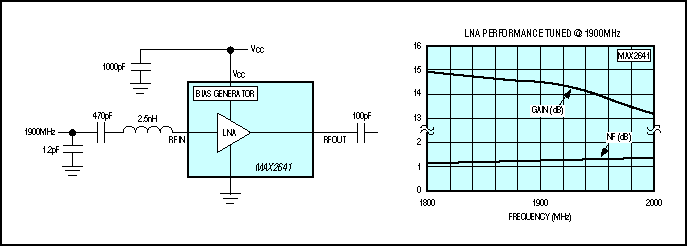# Silicon Germanium (SiGe) Technology Enhances Radio Front-End Performance

### Abstract

This application note describes how silicon germanium enhances IC performance in RF applications. A Giacoleto model is used to analyze noise effects. Wider gain bandwidth of SiGe technology is shown to provide lower noise performance. The impact of SiGe on linearity is explored.

Three parameters are increasingly important for cellular handsets and other digital, portable, wireless communication devices. Low power consumption and lightweight batteries lend autonomy to the device, higher front-end sensitivity increases the reception distance, and greater front-end linearity has a directimpact on the admissible dynamic range. This last parameter is gaining emphasiswith the advent of nonconstant-energy modulation schemes such as π/4DQPSKand 8QAM.

Silicon Germanium (SiGe) is the newest innovation for simultaneously improvingthe power consumption, sensitivity, and dynamic range of a receiver. GST-3 isa new high-speed IC process technology based on silicon germanium (SiGe), whichfeatures a transition figure (fT) of 35GHz. A typical front-end blockdiagram (Figure 1) shows the performance possible with Silicon Germanium technology(1.9GHz) for a combination mixer and low-noise amplifier (LNA).Figure 1. Typical radio input circuitry includes a low-noise amplifier and mixer.

### Noise Performance

The main contribution to noise figure in the down-conversion link is noisecreated by the LNA's first transistor input stage. Noise figure (NF) servesas a figure of merit for networks, to compare noise in the actual network withthat in an ideal noiseless network. The noise factor (F) for an amplifier orother network with power gain (G) equal to G = POUT/PINcan be expressed as:NF is a measure of the degradation in the signal-to-noise ratio (SNR) betweenthe input and output ports of a network, typically expressed in dB: NF = 10log10F.Therefore,

 F = Input SNR/Output SNR = (PIN/NIN)/(POUT/NOUT) = NOUT/(NIN. G)

We are concerned with thermal noise (also called Johnson noise or white noise)and shot noise (also called Schottky noise). A detailed high-frequency equivalentmodel for the bipolar transistor (the Giacoleto model—see Figure 2) helps inunderstanding how this noise is generated. The model also shows how SiGe technology can help reduce the LNA's front-end noise figure.Figure 2. This detailed npn-transistor model (the Giacoleto model) simplifies the analysis of frequency effects.

### Silicon Germanium Thermal and Shot Noise

Within a conducting medium whose temperature is above absolute zero (0°K),the random motion of charge carriers produces random noise-producing voltagesand currents. A rising conductor temperature increases the charge-carrier velocityof these random motions, which increases the noise voltage. The thermal noisegenerated by parasitic base resistance (Rbb´) in a transistor is Vn(f)= 4kTRbb´, where Vn(f) equals the voltage spectral noise density in V²/Hz.The k is Boltzmann's constant (1.38×10-23 Joules/Kelvin),and T is the absolute temperature in degrees Kelvin (°C + 273°).

Shot noise is a consequence of the particle-like nature of charge carriers. DC current flow in a semiconductor is often regarded as constant at every instant, but any current consists of individual electrons and holes. Only the time-average flow of these charge carriers appears as constant current. Any fluctuation in the number of charge carriers produces a random current at that instant, which is known as shot noise.

The spectral noise density for shot noise in the base current is Inb(f) = 2qIb = 2qIc/β , where Inb is the current spectral noise density in I²/Hz, Ib is the base dc-biasing current, q is one electron charge (1.6×10-19 coulombs), and β is the transistor's DC current gain. Thus, the total noise spectral density generated by the transistor's input stage is the sum of the thermal and shot noise:

γn = 4kTRbb´ + RSOURCE 2qIc/β

Maxim's new SiGe process, GST-3, was created as an extension of GST-2 (a bipolar process with a transition frequency of 27GHz) by doping the transistor bases with germanium. The result was an important decrease in Rbb´ and a significant increase in the transistor beta. The combined effect of these two changes is better noise figure for the SiGe transistor (vs. that of a silicon transistor with similar collector current). Typically, the transistor noise figure is expressed as:Because RSOURCE = Vn(f)/Inb(f) gives a minimum noise figure for Si-bipolar as well as Silicon Germanium technology, the full benefits of the SiGe process can be obtained by designing an LNA with source impedance close to this value.

Another important aspect of wireless design is the derating of noise figure vs. frequency. The power gain of a typical transistor is similar to the upper curve in Figure 3. This curve is not surprising, considering the equivalent transistor circuit of Figure 2. In effect, the model is an RC lowpass filter whose gain falls off at 6dB per octave. The maximum theoretical frequency for which the common-emitter current gain (β) is unity (0dB) is called the transition frequency (fT). An LNA's gain (G) depends directly on β, so the derating of noise figure [F = NOUT/(NING)] begins with the rolloff of gain.Figure 3. Silicon Germanium (SiGe) bipolar transistors exhibit high gain and low noise.

To see how the GST-3 SiGe process improves noise figure at high frequencies, consider that adding germanium to the p-silicon base of a transistor reduces the bandgap by 80mV to 100mV across the base, creating a strong electric field between the emitter and collector junctions. By rapidly sweeping electrons from the base into the collector, this electric field reduces the transit time (tb) required for carriers to cross the narrow base. If all other factors are held constant, this reduced tb provides an approximate 30% increase in fT.

For identical-area transistors, the Silicon Germanium device achieves a given fT with one-half to one-third the current required in a GST-2 device. Higher fT reduces high-frequency noise, because the β rolloff occurs at a higher frequency.

### Ultra-low-noise SiGe Amplifier (MAX2641)

The Silicon Germanium MAX2641 offers advantages over silicon-bipolar LNAs, whose NF falls off for frequencies approaching the 2GHz limit (i.e., 1.5dB at 1GHz vs. 2.5dB at 2GHz). High reverse isolation in the SiGe device also allows tuning of the input-matching network without affecting the output matching, and vice versa.

The Silicon Germanium MAX2641 is optimized for operation in the 1400MHz to 2500MHz range, with typical performance that includes 14.4dB gain, -4dBm input IP3 (IIP3), 30dB reverse isolation, and a 1.3dB noise figure at 1900MHz (Figure 4). Available in 6-pin SOT23 packages, it operates from a +2.7V to +5.5V single supply, draws 3.5mA, and is internally biased. The only external components typically required are a two-element input match, input and output blocking capacitors, and a VCC bypass capacitor.Figure 4. Note the very low noise figure for this Silicon Germanium integrated-circuit low-noise amplifier.

### Linearity

In addition to noise and finite bandwidth, communication systems are limited by signal distortion. The system's usefulness depends on its dynamic range (i.e., the signal range it can process with high quality). Dynamic range is dictated by noise figure, whose lower limit is defined by the sensitivity level and whose upper limit is defined by the acceptable maximum level of signal distortion. Achieving the optimum dynamic range involves trade-offs among power consumption, output signal distortion, and the level of input signal with respect to noise.

A typical receiver block diagram (Figure 1) shows the relative importance of noise figure and linearity for the LNA and mixer. Because the LNA input is supplied directly by a very low-level signal from the antenna, its NF is the dominant parameter. For the mixer, fed by an amplified signal from the LNA output, linearity is the dominant parameter.

The output is never an exact replica of the input signal because no transistor is perfectly linear. The output signal always includes harmonics, intermodulation distortion (IMD), and other spurious components. In Figure 5, the second term of the POUT equation is called the second harmonic or second-order distortion, and the third term is called the third harmonic or third-order distortion. Both are characterized by driving the device input with a signal consisting of one tone or two pure sinusoidal tones closely spaced in frequency. Third-order intermodulation distortion for the MAX2681, for example, is characterized with a -25dBm signal consisting of tones at 1950MHz and 1951MHz.Figure 5. A two-tone test characterizes harmonic and intermodulation distortion.

A graphic frequency-domain representation of the POUT equation shows that the output consists of fundamental frequencies ω1 and ω2, second harmonics 2ω1 and 2ω2, third harmonics 3ω1 and 3ω2, the second-order intermodulation product IM2, and the third-order intermodulation product IM3. Figure 5 also shows that in cellular handsets and other systems with narrow-band operating frequencies (i.e., a few tens of megahertz, and less than an octave), only the IM3 spurious signals (2ω1 - ω2) and (2ω2 - ω1) fall within the filter passband. The result can be distortion in the desired signals associated with ω1 and ω2.

In the POUT equation for low levels of output power, coefficient K1A is directly proportional to the input signal amplitude, K2A² is proportional to the square, and K3A3 is proportional to the cube of the input amplitude. Thus, the plot of each on a log scale is a straight line with slope corresponding to the order of the response.

Second- and third-order intercept points are often used as figures of merit. The higher the intercept point, the better the device can amplify large signals. At higher power levels, the output response is compressed and therefore deviates from the response of the fundamental. This deviation point (Figure 6a) is defined as the 1dB com- pression point, and is situated where the output signal compresses by 1dB (G1dB = G - 1dB) with respect to an extrapolation of the linear portion of the curve.

From the MAX2681 data sheet, POUT vs. frequency above 1900MHz shows a -56dBc spurious-free dynamic range (SFDR) relative to IM3 (Figure 6b). The typical operating conditions are PRFIN = -25dBm, IIP3 = 0.5dBm, and conversion gain = 8.4dB. LO-to-IF leakage and other spurious artifacts can be filtered by a narrow-bandpass IF filter, as shown in Figure 1. The MAX2681 (a SiGe double-balanced downconverter) achieves this performance with typical ICC currents of only 8.7mA.Figure 6. This Silicon Germanium double-balanced downconverter provides a low (0.5dBm) IIP3 level (a) and a 56dBc dynamic range (b).

Another downconverter mixer (MAX2680) offers different performance specifications. Available in a miniature 6-pin SOT23 package, it consists of a double-balanced Gilbert-cell mixer with single-ended RF, LO, and IF port connections. Like the MAX2681, it operates from a single +2.7V to +5.5V supply, accepts RF inputs between 400MHz and 2500MHz, and downconverts to IF outputs between 10MHz and 500MHz. Supply current in shutdown mode is typically less than 0.1µA. The LO input is a single-ended broadband port whose typical input VSWR (400MHz to 2.5GHz) is better than 2.0:1.

### Front-end Input Sensitivity

To evaluate the front-end sensitivity achievable using MAX2641/MAX2681 downconverters, consider QPSK modulation with a 4MHz signal bandwidth. To simplify calculations, assume a perfect rectangular input filter. First, a 3dB NF (AntNF) must be added to counteract a 3dB insertion loss caused by the antenna switch and front-end passive filter. Next, a post-LNA filter is added to eliminate distortion (other than IM3 distortion) generated by the LNA. Consider using a filter with 2dB of attenuation and NF for this purpose. At 1900MHz, the post-LNA filter NF adds to the MAX2681's 11.1dB NF:

Total NF = filter NF + mixer NF =
2dB + 11.1dB = 13.1dB

The LNA input needs high NF because it is supplied directly by a very low-level signal from the antenna. The mixer NF is attenuated by LNA gain:

Total NF = LNA NF + (1/GLNA)(NFTOTAL - 1) = 2.054;
NFTOTAL (dB) = 10log2.126 = 3.12dB.

With QPSK modulation and a 10-3 BER, the minimum required ratio of bit energy to noise energy at the antenna input is Eb/No = 6.5dB. The absolute noise floor at +25°C is AbsNfl = -174dBm = 10log(KT), where T = +300°K and K = 1.38 × 10-23. The filter bandwidth in dB is FiltBwth = 10log(4MHz) = 66dB. In Figure 1, the front-end sensitivity for QPSK modulation with 10-3 BER is estimated as:

Input sensitivity = AbsNfl + AntNF + FiltBwth + NFtotal + Eb/No
= -174dBm + 3dB + 66dB + 3.12dB + 6.5dB = -95.38dBm.

### Conclusion

When compared with pure bipolar processes, SiGe provides a lower noise figure vs. frequency for frequencies exceeding 1.0GHz. It also provides lower supply current and higher linearity. Maxim has demonstrated a high-linearity silicon germanium mixer that exhibits a typical IIP3 of 0.5dBm at 1900MHz and a noise figure of 11.1dB (SSB) with conversion gain of 8.4dB, while drawing only 8.7mA of supply current. The higher frequency operation permitted by Silicon Germanium's higher transition frequency (fT) enables applications through 5GHz.

### References

1. Richard Lodge, "Advantages of SiGe for GSM RF Front-Ends." Maxim Integrated, Theale, United Kingdom.
2. Chris Bowick, RF Circuit Designs. (Howard W. Sams, & Co. Inc).
3. Tri T. Ha, Solid-State Microwave Amplifier Design. A Wiley-Interscience publication, 1981, ISBN 0-471-08971-0.